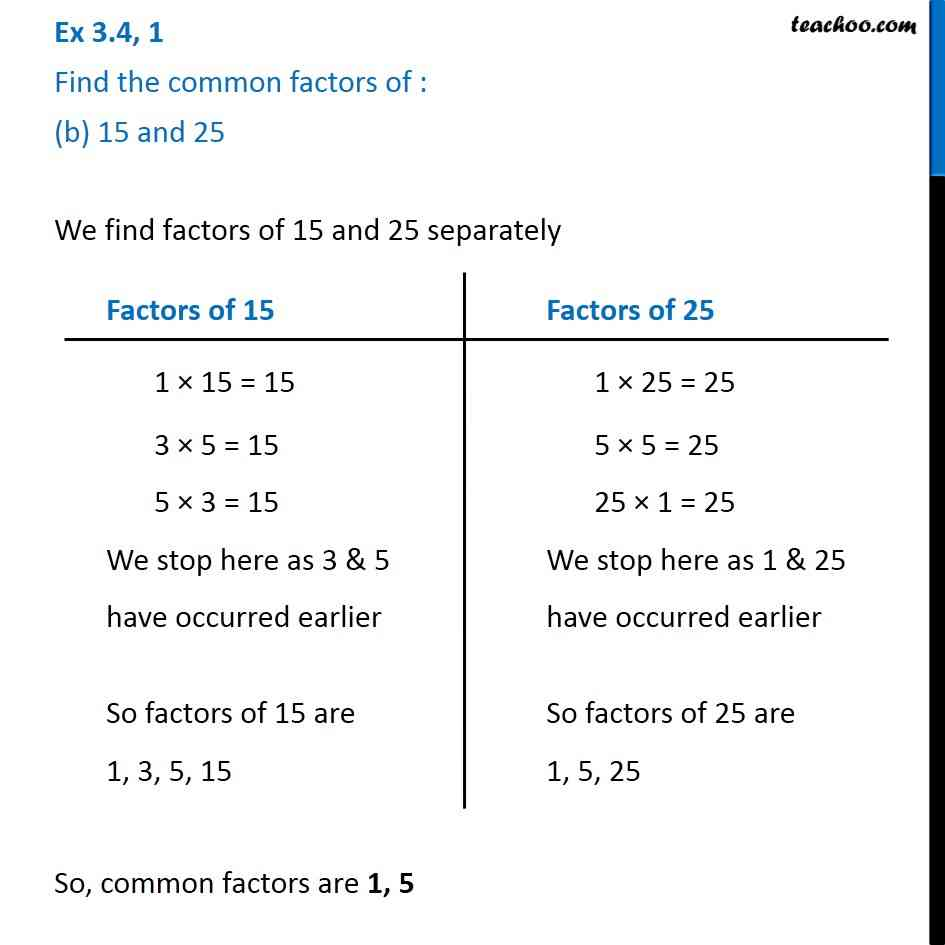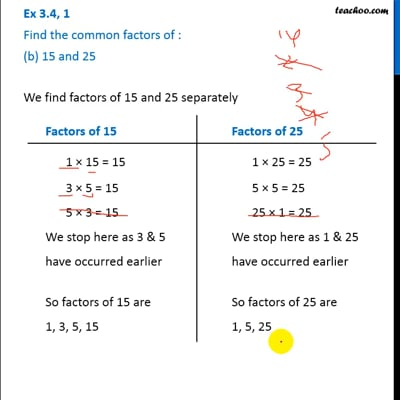Ex 3.4

Chapter 3 Class 6 Playing with Numbers
Serial order wiseThis video is only available for Teachoo black users

Learn in your speed, with individual attention - Teachoo Maths 1-on-1 Class

### Transcript

Ex 3.4, 1 Find the common factors of : (b) 15 and 25 We find factors of 15 and 25 separately So, common factors are 1, 5 Factors of 15 1 × 15 = 15 3 × 5 = 15 5 × 3 = 15 We stop here as 3 & 5 have occurred earlier So factors of 15 are 1, 3, 5, 15 Factors of 25 1 × 25 = 25 5 × 5 = 25 25 × 1 = 25 We stop here as 1 & 25 have occurred earlier So factors of 25 are 1, 5, 25